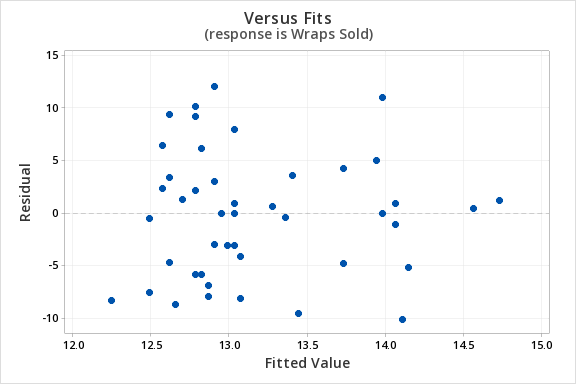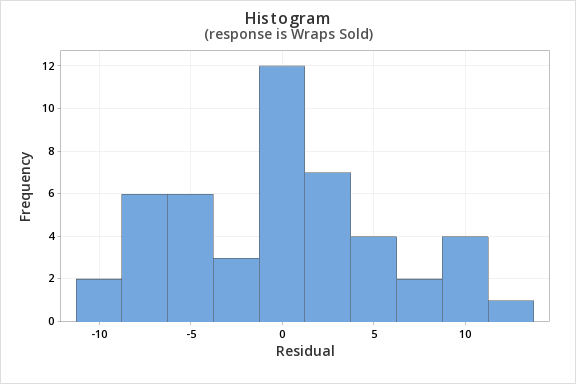# 12.3.4 - Hypothesis Testing

12.3.4 - Hypothesis Testing

We can use statistical inference (i.e., hypothesis testing) to draw conclusions about how the population of $$y$$ values relates to the population of $$x$$ values, based on the sample of $$x$$ and $$y$$ values.

The equation $$Y=\beta_0+\beta_1 x$$ describes this relationship in the population. Within this model there are two parameters that we use sample data to estimate: the $$y$$-intercept ($$\beta_0$$ estimated by $$b_0$$) and the slope ($$\beta_1$$ estimated by $$b_1$$). We can use the five step hypothesis testing procedure to test for the statistical significance of each  separately. Note, typically we are only interested in testing for the statistical significance of the slope because that tells us that $$\beta_1 \neq 0$$ which means that $$x$$ can be used to predict $$y$$. When $$\beta_1 = 0$$ then the line of best fit is a straight horizontal line and having information about $$x$$ does not change the predicted value of $$y$$; in other words, $$x$$ does not help us to predict $$y$$. If the value of the slope is anything other than 0, then the predict value of $$y$$ will be different for all values of $$x$$ and having $$x$$ helps us to better predict $$y$$.

We are usually not concerned with the statistical significance of the $$y$$-intercept unless there is some theoretical meaning to $$\beta_0 \neq 0$$. Below you will see how to test the statistical significance of the slope and how to construct a confidence interval for the slope; the procedures for the $$y$$-intercept would be the same.

1. Check assumptions and write hypotheses

The assumptions of simple linear regression are linearity, independence of errors, normality of errors, and equal error variance. You should check all of these assumptions before preceding.

Research Question Is the slope in the population different from 0? Is the slope in the population positive? Is the slope in the population negative?
Null Hypothesis, $$H_{0}$$ $$\beta_1 =0$$ $$\beta_1= 0$$ $$\beta_1= 0$$
Alternative Hypothesis, $$H_{a}$$ $$\beta_1\neq 0$$ $$\beta_1> 0$$ $$\beta_1< 0$$
Type of Hypothesis Test Two-tailed, non-directional Right-tailed, directional Left-tailed, directional
2. Calculate the test statistic

Minitab will compute the $$t$$ test statistic:

$$t=\dfrac{b_1}{SE(b_1)}$$ where $$SE(b_1)=\sqrt{\dfrac{\dfrac{\sum (e^2)}{n-2}}{\sum (x- \overline{x})^2}}$$

3. Determine the p-value

Minitab will compute the p-value for the non-directional hypothesis $$H_a: \beta_1 \neq 0$$

If you are conducting a one-tailed test you will need to divide the p-value in the Minitab output by 2.

4. Make a decision

If $$p\leq \alpha$$ reject the null hypothesis. If $$p>\alpha$$ fail to reject the null hypothesis.

5. State a "real world" conclusion

Based on your decision in Step 4, write a conclusion in terms of the original research question.

# 12.3.4.1 - Example: Quiz and exam scores

12.3.4.1 - Example: Quiz and exam scores

Construct a model using quiz averages to predict final exam scores.

This example uses the exam data set found in this Minitab file: Exam.mpx

1. Check assumptions and write hypotheses
• $$H_0\colon \beta_1 =0$$
• $$H_a\colon \beta_1 \neq 0$$

The scatterplot below shows that the relationship between quiz average and final exam score is linear (or at least it's not non-linear).The plot of residuals versus fits below can be used to check the assumptions of independent errors and equal error variances. There is not a significant correlation between the residuals and fits, therefore the assumption of independent errors has been met. The variance of the residuals is relatively consistent for all fitted values, therefore the assumption of equal error variances has been met.Finally, we must check for the normality of errors. We can use the normal probability plot below to check that our data points fall near the line. Or, we can use the histogram of residuals below to check that the errors are approximately normally distributed.Now that we have check all of the assumptions of simple linear regression, we can examine the regression model.

2. Calculate the test statistic
We will use the coefficients table from the Minitab output.
##### Coefficients
Term Coef SE Coef T-Value P-Value VIF
Constant 12.1 11.9 1.01 0.315
Quiz_Average 0.751 0.141 5.31 0.000 1.00
$$t = 5.31$$
3. Determine the p-value

$$p=0.000$$

4. Make a decision

$$p < \alpha$$, reject the null hypothesis

5. State a "real world" conclusion

There is evidence that that students' quiz averages can be used to predict their final exam scores in the population.

# 12.3.4.2 - Example: Business Decisions

12.3.4.2 - Example: Business Decisions

A student-run cafe wants to use data to determine how many wraps they should make today. If they make too many wraps they will have waste. But, if they don't make enough wraps they will lose out on potential profit. They have been collecting data concerning their daily sales as well as data concerning the daily temperature. They found that there is a statistically significant relationship between daily temperature and coffee sales. So, the students want to know if a similar relationship exists between daily temperature and wrap sales. The video below will walk you through the process of using simple linear regression to determine if the daily temperature can be used to predict wrap sales.  The screenshots and annotation below the video will walk you through these steps again.

Can daily temperature be used to predict wrap sales?

Data concerning sales at a student-run cafe were obtained from a Journal of Statistics Education article. Data were retrieved from cafedata.xls more information about this data set available at cafedata.txt.

For the analysis you can use the Minitab file: cafedata.mpx

1. Check assumptions and write hypotheses
• $$H_0\colon \beta_1 =0$$
• $$H_a\colon \beta_1 \neq 0$$

The scatterplot below shows that the relationship between maximum daily temperature and wrap sales is linear (or at least it's not non-linear). Though the relationship appears to be weak.The plot of residuals versus fits below can be used to check the assumptions of independent errors and equal error variances. There is not a significant correlation between the residuals and fits, therefore the assumption of independent errors has been met. The variance of the residuals is relatively consistent for all fitted values, therefore the assumption of equal error variances has been met.Finally, we must check for the normality of errors. We can use the normal probability plot below to check that our data points fall near the line. Or, we can use the histogram of residuals below to check that the errors are approximately normally distributed.Now that we have check all of the assumptions of simple linear regression, we can examine the regression model.

2. Calculate the test statistic
From Minitab...
##### Regression Equation

Wraps Sold = 11.42 + 0.0414 Max Daily Temperature (F)

##### Coefficients
Term Coef SE Coef T-Value P-Value VIF
Constant 11.42 2.66 4.29 0.000
Max Daily Temperature (F) 0.0414 0.0603 0.69 0.496 1.00
##### Model Summary
S R-sq R-sq(adj) R-sq(pred)
5.90208 1.04% 0.00% 0.00%
##### Analysis of Variance
Source DF Adj SS Adj MS F-Value P-Value
Regression 1 16.41 16.41 0.47 0.496
Max Daily Temperature (F) 1 16.41 16.41 0.47 0.496
Error 45 1567.55 34.83
Lack-of-Fit 24 875.17 36.47 1.11 0.411
Pure Error 21 692.38 32.97
Total 46 1583.96

$$t = 0.69$$

3. Determine the p-value

$$p=0.496$$

4. Make a decision

$$p > \alpha$$, fail to reject the null hypothesis

5. State a "real world" conclusion

There is not evidence that maximum daily temperature can be used to predict the number of wraps sold in the population of all days.

  Link ↥ Has Tooltip/Popover Toggleable Visibility